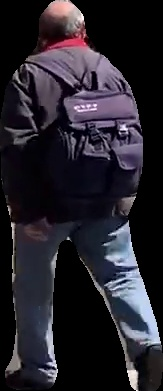# Instance segmentation of videos with PixelLib¶

Instance segmentation with PixelLib is based on MaskRCNN framework.

Code to implement instance segmentation of videos:

```import pixellib
from pixellib.instance import instance_segmentation

segment_video = instance_segmentation()
segment_video.process_video("video_path", frames_per_second= 20, output_video_name="path_to_outputvideo")
```
```import pixellib
from pixellib.instance

segment_video = instance_segmentation()
```

We imported in the class for performing instance segmentation and created an instance of the class.

```segment_video.load_model("mask_rcnn_coco.h5")
```

```segment_video.process_video("sample_video2.mp4", frames_per_second = 20, output_video_name = "output_video.mp4")
```

We called the function to perform segmentation on the video file.

It takes the following parameters:-

video_path: the path to the video file we want to perform segmentation on.

frames_per_second: this is parameter to set the number.of frames per second for the output video file. In this case it is set to 15 i.e the saved video file will have 15 frames per second.

output_video_name: the saved segmented video. The output video will be saved in your current working directory.

Sample video2

```import pixellib
from pixellib.instance import instance_segmentation

segment_video = instance_segmentation()
segment_video.process_video("sample_video2.mp4", frames_per_second= 15, output_video_name="output_video.mp4")
```

Output video

We can perform instance segmentation with object detection by setting the parameter show_bboxes to true.

```import pixellib
from pixellib.instance import instance_segmentation

segment_video = instance_segmentation()
segment_video.process_video("sample_video2.mp4", show_bboxes = True, frames_per_second= 15, output_video_name="output_video.mp4")
```

Output video with bounding boxes

# Extraction of objects in videos¶

PixelLib supports the extraction of objects in videos and camera feeds.

```segment_video.process_video("sample.mp4", show_bboxes=True,  extract_segmented_objects=True,save_extracted_objects=True, frames_per_second= 5,  output_video_name="output.mp4")
```

It still the same code except we introduced new parameters in the process_video which are:

extract_segmented_objects: this is the parameter that tells the function to extract the objects segmented in the image. It is set to true.

save_extracted_objects: this is an optional parameter for saving the extracted segmented objects.

Extracted objects from the video# Segmentation of Specific Classes in Videos¶

The pre-trained coco model used detects 80 classes of objects. PixelLib has made it possible to filter out unused detections and detect the classes you want.

```target_classes = segment_video.select_target_classes(person=True)
segment_video.process_video("sample.mp4", show_bboxes=True, segment_target_classes= target_classes, extract_segmented_objects=True,save_extracted_objects=True, frames_per_second= 5,  output_video_name="output.mp4")
```

The target class for detection is set to person and we were able to segment only the people in the video.

```target_classes = segment_video.select_target_classes(car = True)
segment_video.process_video("sample.mp4", show_bboxes=True, segment_target_classes= target_classes, extract_segmented_objects=True,save_extracted_objects=True, frames_per_second= 5,  output_video_name="output.mp4")
```

The target class for detection is set to car and we were able to segment only the cars in the video.

Full code for filtering classes and object Extraction

# Instance Segmentation of Live Camera with Mask R-cnn.¶

We can use the same model to perform semantic segmentation on camera. This can be done by few modifications to the code used to process video file.

```import pixellib
from pixellib.instance import instance_segmentation
import cv2

capture = cv2.VideoCapture(0)

segment_video = instance_segmentation()
segment_video.process_camera(capture, frames_per_second= 15, output_video_name="output_video.mp4", show_frames= True,
frame_name= "frame")
```
```import cv2
capture = cv2.VideoCapture(0)
```

We imported cv2 and included the code to capture camera frames.

```segment_video.process_camera(capture, show_bboxes = True, frames_per_second = 15, output_video_name = "output_video.mp4", show_frames = True, frame_name = "frame")
```

In the code for performing segmentation, we replaced the video filepath to capture i.e we are going to process a stream camera frames instead of a video file.We added extra parameters for the purpose of showing the camera frames.

show_frames this parameter handles showing of segmented camera frames and press q to exist.

frame_name this is the name given to the shown camera’s frame.

A demo showing the output of pixelLib’s instance segmentation of camera’s feeds using Mask-RCNN. Good work! It was able to successfully detect me and my phone.

Detection of Target Classes in Live Camera Feeds

This is the modified code below to filter unused detections and detect a target class in a live camera feed.

```import pixellib
from pixellib.instance import instance_segmentation
import cv2

capture = cv2.VideoCapture(0)

segment_video = instance_segmentation()
target_classes = segment_video.select_target_classes(person=True)
segment_video.process_camera(capture, segment_target_classes=target_classes,  frames_per_second= 10, output_video_name="output_video.mp4", show_frames= True,frame_name= "frame")
```

Full code for Filtering classes and object Extraction in Camera feeds

```import pixellib
from pixellib.instance import instance_segmentation
import cv2

capture = cv2.VideoCapture(0)

segment_video = instance_segmentation()
target_classes = segment_video.select_target_classes(person=True)
segment_video.process_camera(capture, segment_target_classes=target_classes,  frames_per_second= 10, extract_segmented_objects=True,
save_extracted_objects=True,output_video_name="output_video.mp4", show_frames= True,frame_name= "frame")
```

PixelLib now supports the ability to adjust the speed of detection according to a user’s needs. The inference speed with a minimal reduction in the accuracy of detection. There are three main parameters that control the speed of detection.

1 average

2 fast

3 rapid

By default the detection speed is about 1 second for a processing a single image using Nvidia GeForce 1650.

Using Average Detection Mode

```import pixellib
from pixellib.instance import instance_segmentation
import cv2

capture = cv2.VideoCapture(0)

segment_video = instance_segmentation(infer_speed = "average")
segment_video.process_camera(capture, frames_per_second= 10, output_video_name="output_video.mp4", show_frames= True,
frame_name= "frame")
```

In the modified code above within the class instance_segmentation we introduced a new parameter infer_speed which determines the speed of detection and it was set to average. The average value reduces the detection to half of its original speed, the detection speed would become 0.5 seconds for processing a single image.

Using fast Detection Mode

```import pixellib
from pixellib.instance import instance_segmentation
import cv2

capture = cv2.VideoCapture(0)

segment_video = instance_segmentation(infer_speed = "fast")
segment_video.process_camera(capture, frames_per_second= 10, output_video_name="output_video.mp4", show_frames= True,
frame_name= "frame")
```

In the code above we replaced the infer_speed value to fast and the speed of detection is about 0.35 seconds for processing a single image.

Using rapid Detection Mode

```import pixellib
from pixellib.instance import instance_segmentation
import cv2

capture = cv2.VideoCapture(0)

segment_video = instance_segmentation(infer_speed = "rapid")